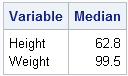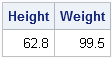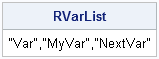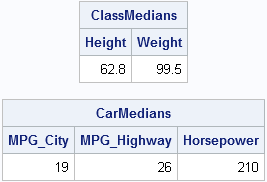When I call R from within the SAS/IML language, I often pass parameters from SAS into R. This feature enables me to write general-purpose, reusable, modules that can analyze data from many different data sets.

I've previously blogged about how to pass values to SAS procedures from PROC IML by using the SAS/IML SUBMIT and ENDSUBMIT statements. You might be surprised to learn that the same technique works when calling R from the SAS/IML language.

To keep things simple, let's focus on a specific statistical example. Suppose that I want to compute the sample medians for several variables in several data sets. In Base SAS, I can compute medians by calling the MEANS procedure as follows:

```proc means data=Sashelp.Class median maxdec=1; var Height Weight; run;```If I want to create a reusable function that perform this analysis on arbitrary variables for an arbitrary data set, I could define a SAS macro named %MEDIAN that takes name of the data set and list of variables. Those parameters are substituted into the PROC MEANS syntax. For example, after defining the macro I could compute the medians of variables in the Sashelp.Cars data set with minimal typing:

`%MEDIAN(data=Sashelp.Cars, vars=MPG_City MPG_Highway Horsepower)`

This article shows how to write a reusable SAS/IML module that calls R to compute the medians for arbitrary variables in an arbitrary SAS data set. Yes, calling R to compute medians is "overkill." PROC MEANS, PROC UNIVARIATE, and the MEDIAN function in SAS/IML all provide this computation. However, the technique used in this simple example (namely, passing parameters from SAS to R) extends to more complicated analyses.

### A basic call to R from SAS/IML

First, let's review how to call R from the SAS/IML language. The typical steps are as follows:

1. Use the ExportDatasetToR subroutine to transfer data from a SAS data set to an R data frame.
2. Call R by using the SUBMIT and ENDSUBMIT statements.
3. Optionally, retrieve the results by using the ImportMatrixFromR subroutine. (Or use the ImportDatasetFromR subroutine to create a SAS data set.)

The following program calls R to compute the medians of two variables in the Sashelp.Class data set. In the R language, you can compute the medians of several variables by using the apply function:

```proc iml; run ExportDatasetToR("Sashelp.Class", "Class"); submit / R; varnames <- c("Height","Weight") m <- apply( Class[varnames], 2, median ) endsubmit; run ImportMatrixFromR(Medians, "m"); print (Medians`)[c={"Height" "Weight"}];```Now suppose that you want to repeat this task many times on different data. It would be helpful to write a SAS/IML function that calls R to compute the medians of specified variables. For example, you might want to call the function as follows:

`ClassMedians = MedianInR("Sashelp.Class", {"Height" "Weight"}); /* GOAL */`

The function will encapsulate the process of calling R. It will transfer the data to R, pass the variable names to R, tell R to compute the medians, and retrieve the results.

### Passing arguments from SAS to R

The key to the technique is to pass parameters to R by using the SUBMIT statement. If you include the name of a SAS/IML vector on the SUBMIT statement, the contents of that vector are substituted for the expression before the SUBMIT block is sent to R. The following statements illustrate passing parameters to R:

```Dataset = "Class"; /* 1st parameter to pass */ RVarList = '"Height","Weight"'; /* 2nd parameter */ submit Dataset RVarList / R; varnames <- c(&RVarList) # expand 1st parameter value m <- apply( &Dataset[varnames], 2, median ) # expand 2nd parameter value endsubmit;```

R does not have the concept of macro variables, so although the expressions &RVarList and &Dataset look like they are macro expression, in reality the program substitutes the contents of those SAS/IML matrices at run time. Also, notice that I want R to receive a comma-separated list of quoted strings, so I used single quotes in SAS to make a string that contains double-quoted variable names.

### Creating a list of variable names from a SAS/IML vector

Most likely the names of the variables are going to be contained in a SAS/IML vector, but you can use standard string concatenation in SAS to form the RVarList string from a vector of variable names. The following SAS/IML statements create a comma-separated list of double-quoted strings:

```/* make a string like '"Var","MyVar","NextVar"' */ VarList = {"Var" "MyVar" "NextVar"}; RVarList = strip(rowcatc('"' + rowvec(Varlist) + '",')); RVarList = substr(RVarList, 1, nleng(RVarList)-1); print RVarList;```### Writing a reusable SAS/IML module that calls R

All the pieces are now in place. You can form a comma-separated list of quoted variable names from values in a SAS/IML vector. You can use the SUBMIT statement to pass that string and the name of the SAS data set to R. R can compute the desired statistics, and then you can retrieve the results. The following statements define a SAS/IML module that implements the algorithm:

```start MedianInR( dataset, VarList ); run ExportDatasetToR(dataset, dataset); /* make a string like '"Var","MyVar","NextVar"' */ RVarList = strip(rowcatc('"' + rowvec(Varlist) + '",')); RVarList = substr(RVarList, 1, nleng(RVarList)-1); /* pass values from SAS to R */ submit dataset RVarList / R; varnames <- c(&RVarList) m <- apply( &dataset[varnames], 2, median ) endsubmit; run ImportMatrixFromR(result, "m"); /* retrieve the result */ return(result`); finish;```

You can now call the module on any SAS data set that will fit into RAM. (Recall that R must hold the complete data frame in memory.)

```VarNames = {"Height" "Weight"}; ClassMedians = MedianInR("Sashelp.Class", VarNames); print ClassMedians[c=VarNames];   VarNames = {"MPG_City" "MPG_Highway" "Horsepower"}; CarMedians = MedianInR("Sashelp.Cars", VarNames); print CarMedians[c=VarNames];```Success! The process of passing parameters to R is very easy. This example was complicated by the need to pass a comma-separated list of quoted variable names to R. Sorry about that, but sometimes tricks like this are necessary when passing parameters to R.

In conclusion, by using the SUBMIT statement in the SAS/IML language, you can pass parameters from SAS to R. This enables you to write general-purpose, reusable functions that call R to compute a statistical analysis. You can use this technique to seamlessly incorporate R computations into a larger SAS program.

ShareDistinguished Researcher in Computational Statistics

Rick Wicklin, PhD, is a distinguished researcher in computational statistics at SAS and is a principal developer of SAS/IML software. His areas of expertise include computational statistics, simulation, statistical graphics, and modern methods in statistical data analysis. Rick is author of the books Statistical Programming with SAS/IML Software and Simulating Data with SAS.

1.•```submit / R; options(stringsAsFactors = FALSE) endsubmit;```